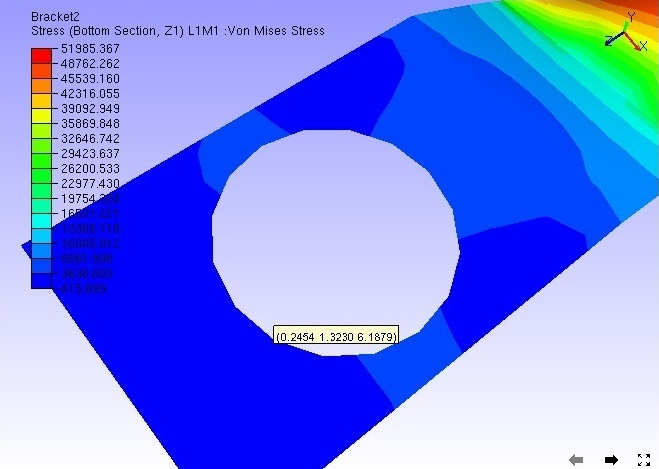# Measure

The Measure option found in the context menu lets users measure the following:

• Linear Measurement

• Point to Point

• Point to Edge

• Point to Face

• Arc Measurement

• Snap Vertex

• Vertex Info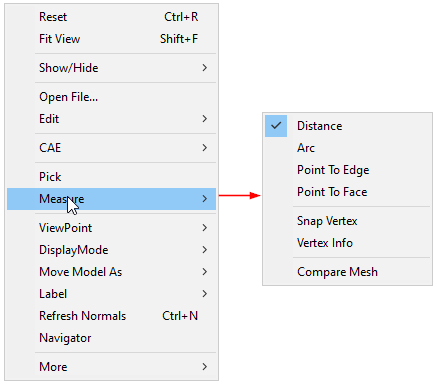Linear Measurement

How to measure point to point?

• Select Measure | Distance option from the Viewer context Menu or click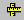from the toolbar.

• Click 2 points of interest on the model to measure the distance.

• The points clicked are highlighted in red and a line is drawn between the endpoints.

• An annotated label with distance value is attached to the middle point of the line drawn.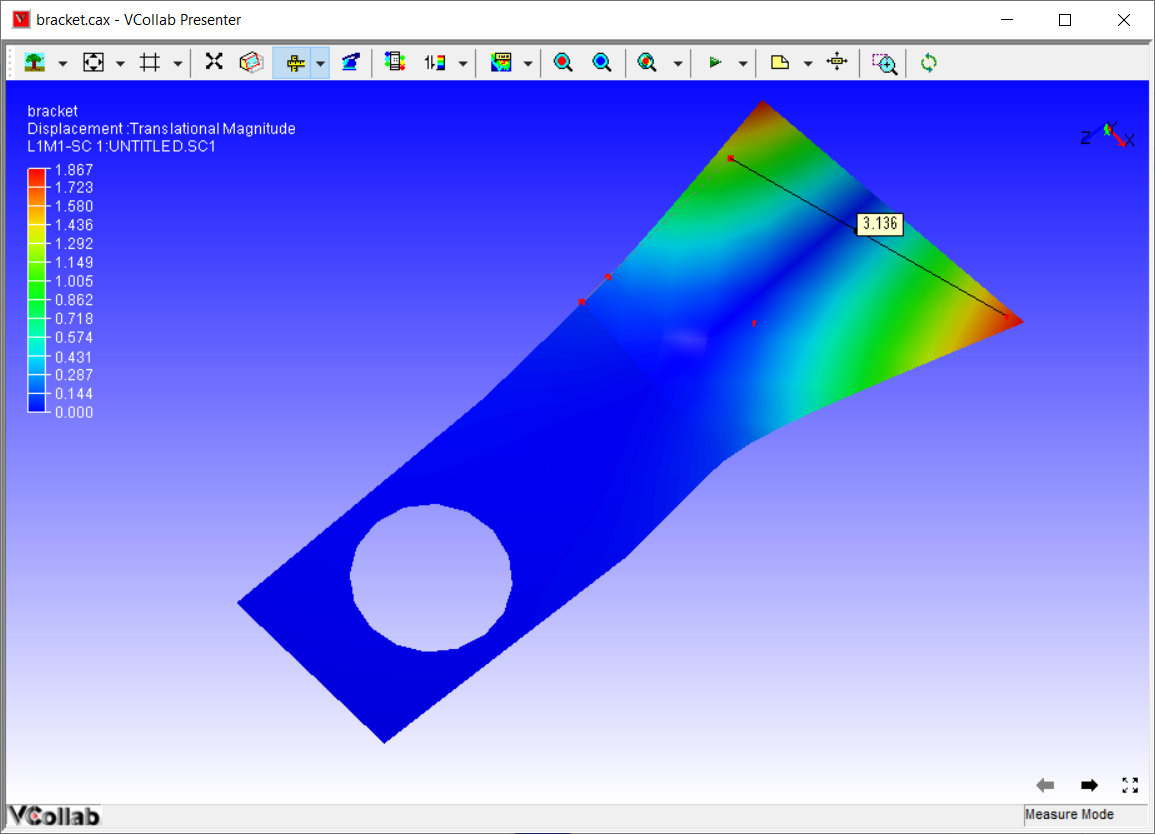How to measure point to edge?

• Click Measure | Point to Edge option from the context menu.

• Click a point on a model and click on the closest triangle edge.

• The point and the closest edge will be highlighted in red. Edge is extended if the foot of perpendicular does not lie in the edge segment.

• An annotated label with distance value is attached to the middle point of the line drawn.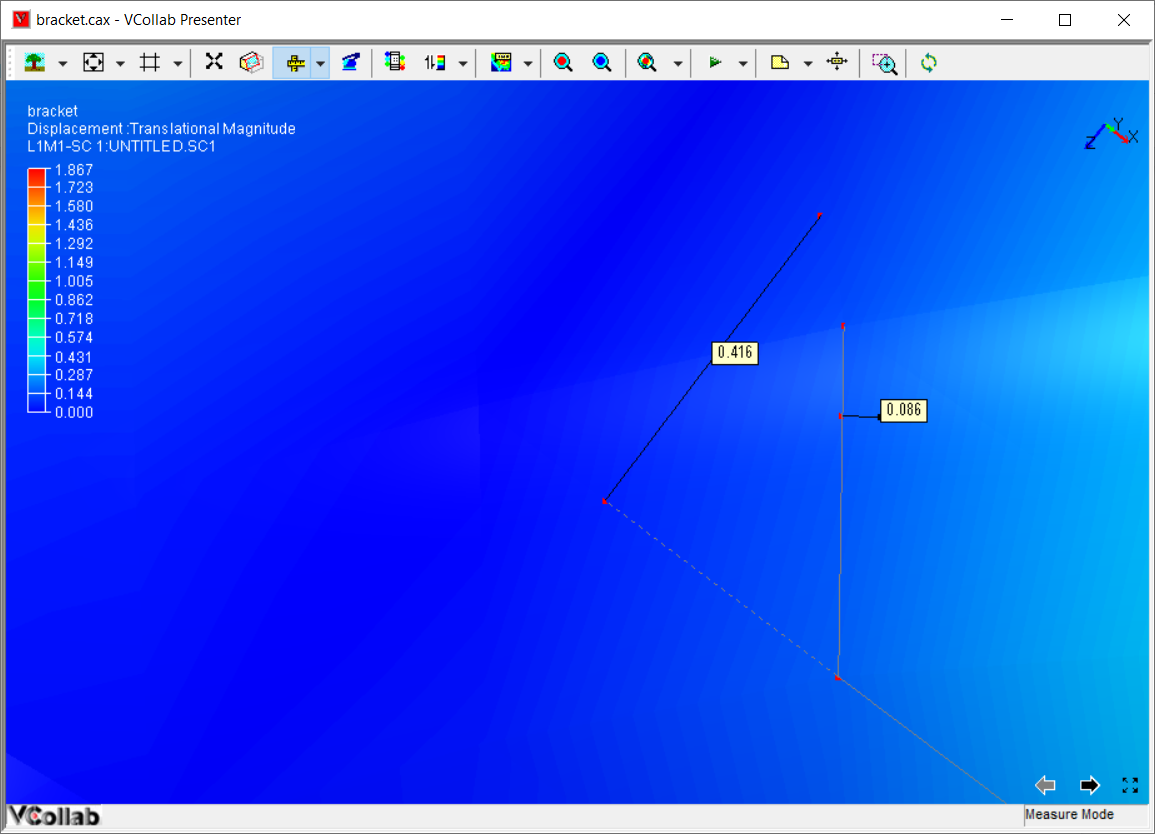How to measure point to face

• Click Measure | Point to Face option from the context menu

• Click a point and a triangle on the model

• The point and triangle points will be highlighted in red.

• An annotated label with distance value is attached to the middle point of line drawn.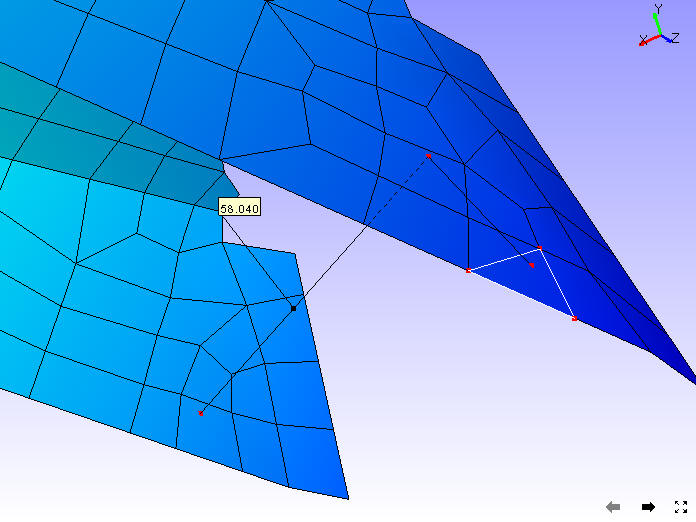How to measure an arc ?

• Click Measure | Arc option from the context menu.

• Click on 3 points of interest to create Arc

• An arc is drawn from start point to end point passing through the second point.

• All three points are highlighted in red.

• An arrow is drawn from the center of the arc to the curve to denote the radius.

• An annotated label with arc information is attached to the center of the arc.

• The part of the curve that is hidden behind the model is drawn with dashed lines.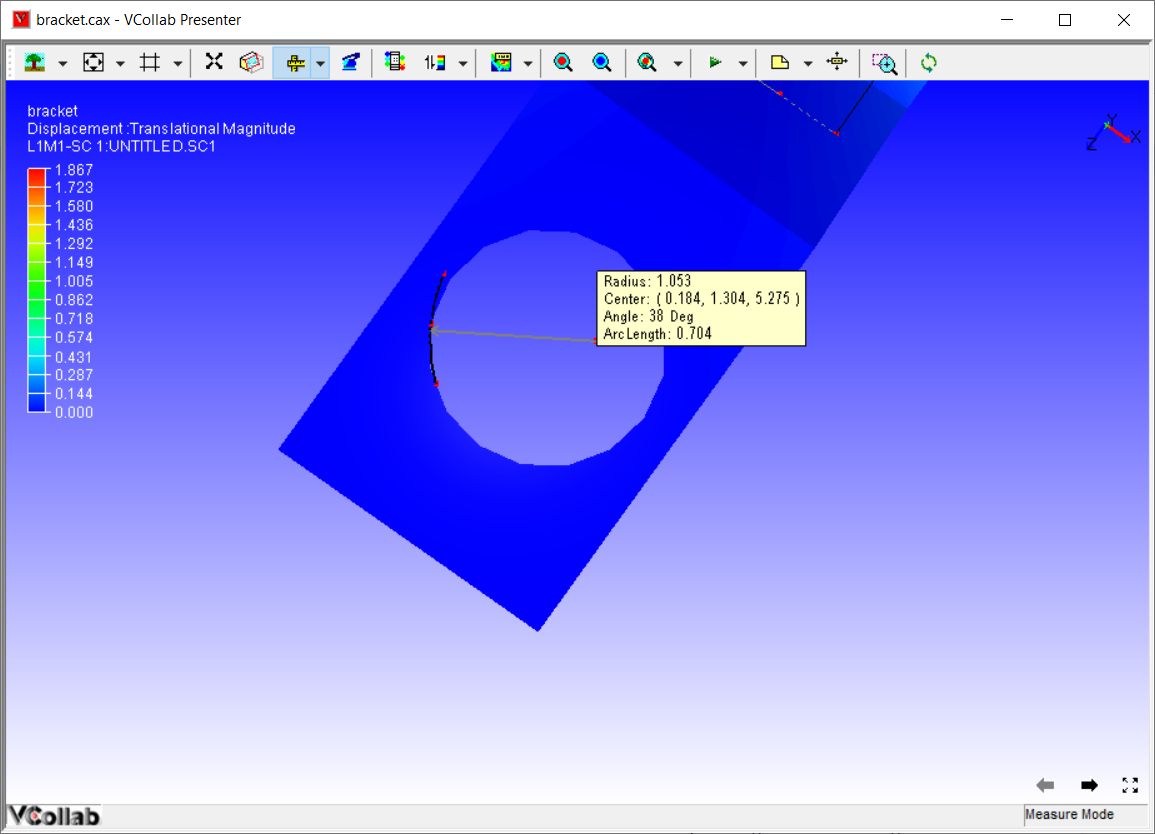How to use the Snap Vertex option?

• This enables the user to select the nearest vertex of the model by clicking while measuring distance or an arc.

• Users can use the Snap Vertex option to assure that the arc computed and drawn is passing through actual arc points of the model.

How to use the Vertex Info option?

When this option is selected, users can see a label with the X, Y, Z coordinates of an existing vertex, while moving the mouse on the model.